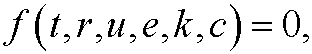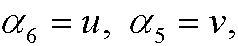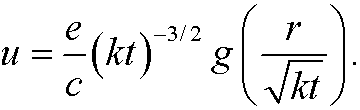# 6. One more example.

We consider a typical heat conduction problem. At time t=0 we let an amount of heat e concentrated in a point in space diffuse outward in a region with original temperature zero.If r denotes the radial distance from the source and t is the time, then the problem consists of determine the temperature u as a function of r and t. We easily realize that the temperature u depends on t, r and e. It is also reasonable that it depends on the heat capacity c of the region as well as the speed with which the heat diffuses outward (the heat diffusivity k). We therefore assume a physical law on the formrelating the fundamental quantities t, r, u, e, k, c. These quantities have the dimensions

 Symbol: Quantity: Dimension: t time T r length L u temperaturee energy E k heat diffusivity L2/T c heat capacityThe dimension matrix A then becomesWe thus have m=6 (number of physical quantities), n=4 (number of fundamental dimensions) and r=4 (rank of the dimension matrix). This implies that we can construct m-r=6-2=2 dimensionless quantities. We therefore look for two linearly independent solutions of the systemIf we letwe getthat isIf we choose v=-1/2 and u=0, we get one solutionIf we choose u=1 and v=3/2, we get another (linearly independent) solutionThese two solutions give the two dimensionless variablesAccording to the Pi-theorem we then conclude that there is an equivalent relationthat isIf we finally solve for u, we get